Courses

# Adsorption Introduction (Part - 1) Chemistry Notes | EduRev

## Chemistry : Adsorption Introduction (Part - 1) Chemistry Notes | EduRev

The document Adsorption Introduction (Part - 1) Chemistry Notes | EduRev is a part of the Chemistry Course Physical Chemistry.
All you need of Chemistry at this link: Chemistry

1. Introduction:

The term adsorption implies the presence of excess concentration of any particular component at the surface of liquid or solid phase as compared to that present in the bulk of the material. This phenomenon of adsorption is basically due to the presence of residual forces at the surface of the body. These residual forces, in case of liquids, arise from the non-uniform distribution of molecules around the molecules at the surface. In solids, these residual forces are due to the presence of unsatisfied valence force of atom at the surface.

The phenomenon of adsorption is a spontaneous process and hence, like any other spontaneous process, is attained by a decrease in free energy of the system, i.e. ΔG of the adsorption process has a negative value. Since ΔG = ΔH – TΔS and that the entropy change ΔS for adsorption is necessarily a negative quantity.

In may be concluded that the enthalpy change of the adsorption process must have a negative value and must satisfy the relation. |ΔH | > |TΔS|

Hence, the process of adsorption is an exothermic process. This also follows directly from the fact that the adsorption process involves the forces of attraction between the adsorbate (the substance which is being adsorbed) and the adsorbent (the substance which adsorbed the adsorbate) and hence on adsorption, there must be a release of energy.

2. Distinction between Adsorption and Absorption

In adsorption the concentration of the adsorbate increases only at the surface of the adsorbent, while in absorption the concentration is uniform throughout the bulk of the solid.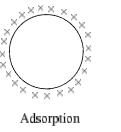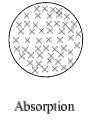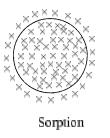Adsorption is due to the face that the surface particles of the adsorbent are different state than the particles inside the bulk. Inside the adsorbent all the force acting between the particles are mutually balanced but on the surface the particles are not surrounded by atoms or molecules of their kind on all sides and hence they possess unbalanced or residual attractive forces. These forces of the adsorbent are responsible for attracting the adsorbate particle on its surface.

3. Types of Adsorption

There are two main types of adsorption of gases on solids.

If accumulation of gas on the surface of a solid occurs on account of weak van der Walls’ force, the adsorption is termed as physical adsorption or physic-sorption.

When the gas molecules or atoms are held to the solid surface by chemical bonds, the adsorption is termed chemical adsorption or chemisorption. The chemical bonds may be covalent or ionic in natureChemisorption has a rather high energy of activation and is, therefore, often referred to as activated adsorption. Sometimes these two processes occur simultaneously and it is not easy to ascertain the type of adsorption. A physical adsorption at low temperature may pass into chemisorption as the temperature is increased. For example, hydrogen is first adsorbed on nickel by van der Walls’ force. Molecules of hydrogen then dissociate and hydrogen atoms are held on the surface by chemisorption.

4. Comparison of Physi-Sorption and Chemisorption

On the basis of forces of attraction between adsorbent and adsorbate, adsorption has been classified into two categories, namely, van der Waals adsorption (or physical adsorption) and chemisorption (or activated adsorption).

 S. No. Physical adsorption Chemical adsorption 1 It is caused by intermolecular van der Waals’ forces. It is caused by chemical bond formation 2 It is not specific It is highly specific 3 It is reversible It is irreversible 4 It depends on the nature of gas. More easily liquefiable gases are adsorbed readily. It depends on the nature of gas. Gases which form compounds with the adsorbent exhibit chemisorption. 5 Heat of adsorption is low. Heat of adsorption is high 6 Low temperature is favourable. It decreases with increases of temperature. High temperature is favourable. It increases with increase of temperature. 7 No appreciable activation energy is involved. High activation energy is involved. 8 High pressure is favourable. Decrease of pressure causes desorption. High pressure is favourable. Decrease of pressure does not cause desorption. 9 It depends on the surface area. It increases with increase of surface area. It also depends on the surface area. It increases with increase of surface area. 10 If forms multilayers on adsorbent surface under high pressure. it forms unimolecular layer.

5. Adsorption of Gases on Solids

Following are the factors which influence the extent of adsorption of gases on solid surface:

(a) Nature of adsorbent:

• Transition metals are good adsorbent for gases because of vacant or half filled d-orbitals and high charge-size ratio.
• Activated charcoal (a good adsorbent for poisonous and polluting gases in gas mask)
• Metal oxides such as silica gel (a good adsorbent for moisture) and aluminium oxide, clay are other good adsorbent.

(b) Nature of adsorbate: Easily liquefiable gases (e.g., HCl, NH3, CO2) are adsorbed to greater extent than others (e.g., H2, N2, O2). The adsorption  of gases on finely divided metal surface is also called occlusion.

(c) Surface area of adsorbent:  Larger is surface area of adsorbent more is adsorption. The surface area per gram of adsorbent is called specific surface area of adsorbent.

That is why a porous or finely divided form of adsorbents adsorb larger quantities of adsorbate.

(d) Activation of solid adsorbent: The activation of surface for adsorption can be made by rubbing or by passing in colloidal state or by passing super heated steam, etc. Activated charcoal is commonly used for the adsorption of easily liquefiable gases. Also the active sites on adsorbent can be increased by heating the adsorbent in vacuum.

(e) Temperature: An increase in temperature decreases physical adsorption (Figure 1) whereas, chemisorption first increases and then decreases. Usually adsorption being exothermic and thus effect of temperature is in accordance with Le-Chatelier principle. However in chemisorption, some activation energy is needed to cross the energy barrier of adsorption like ordinary chemical reactions. The plots of extent of adsorption (x/m) vs. temperature at constant pressure, are called adsorption isobars.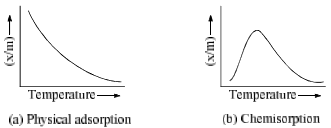(f) Pressure: The plots of extent of adsorption (x/m) vs. pressure at constant temperature are called adsorption isotherms, ‘x’ is the amount of adsorbate on m gram of adsorption. Freundlich studied the influence of pressure on adsorption of gases at different temperature and obtained Figure. 2.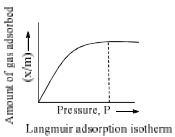6. Desorption Activation Energy: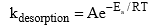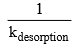is called life time, τ of the adsorbate molecule on the surface, therefore,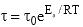where,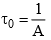is known as the pre-exponential factors and Ea = desorption activation energy or adsorbate.

7. Freundlich Equation

Freundlich, in 1909, gave an empirical relationship between the quantity of gas adsorbed by unit mass of solid adsorbent and pressure at a particular temperature. The relationship can be expressed by the following equation.

x/m = kPl/n   (0 < 1/n < 1)

where x is the quantity adsorbed, m is the mass of the adsorbent, P is the pressure of adsorbate and k and n are empirical constant for each adsorbent-adsorbate pair at a given temperature. The function has an asymptotic maximum as pressure increases without bound. As the temperature increases, the constant k and n change to reflect the empirical observation that the quantity adsorbed rises more slowly and higher pressures and required to saturate the surface. The value of k and n for a given system can be determined by following the graphical method. If we take the logarithm of Eq. (1), we get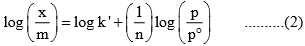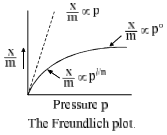Where p° is the unit pressure. A plot of log (x/m) versus log (p/p°) would be a straight line with slope equal to (1/n) and intercept equal to log k’. From these, the values of k and n can be determined.

8. Langmuir Equation

It is based on four assumptions:

1. The surface of the adsorption is uniform, that is, all the adsorption sites are equivalent.

2. Adsorbed molecules do not interact.

3. All adsorption occurs through the same mechanism.

4. At the maximum adsorption, only a monolayer is formed: molecules of adsorbate do not deposit on other, already adsorbed, molecules of adsorbate, only on the free surface of the adsorbent.

Langmuir, suggested that adsorption takes place though this mechanism: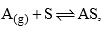where A is a gas molecules, and S is an adsorption site. The direct and inverse rate constants are k and k–1. If we define surface coverage, as the fraction of the adsorption sites occupied, in the equilibrium we have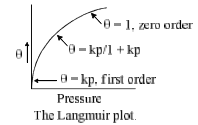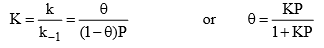Where P is the partial pressure of the gas or the molar concentration of the solution. For very low pressure θ ≈ 1

• The equilibrium constant K° can be utilized for calculating the change in standard free energy on adsorption by the relation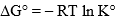The STP volume of adsorbate required to form a monolayer on the adsorbent (per gram of adsorbent).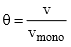and we obtain an expression for a straight line: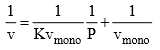Through its slope and y-intercept we can obtain vmono and K, which are constants for each adsorbent/adsorbate pair at a given temperature vmono is related to the number of adsorption sites through the ideal gas law. If we assume that the number of sites is just the whole area of the solid divided into the cross section of the adsorbate molecules, we can easily calculate the surface area of the adsorption.

The surface area of an adsorbent depends on its structure; the more pores it has, the greater the area, which has a big influence on reactions on surfaces.

If more than one gas adsorbs on the surface,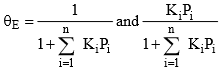Where i is each one of the gases that adsorb.

9. BET adsorption isotherm:

The derivation of the formula is more complicated than Langmuir’s. We obtain: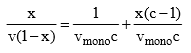x is the pressure divided by the vapour pressure for the adsorbate at that temperature (usually denoted P/P0), v is the STP volume of adsorbate, vmono is the STP volume  of the amount of adsorbate required to form a monolayer and c is the equilibrium constant K we used in Langmuir isotherm multiplied by the vapour pressure of the adsorbate. The key assumption used in deriving the BET equation is that the successive heats of adsorption for all layers except the first are equal to the heat of condensation of the adsorbate.

Illustration: The data below are for the adsorption of CO on charcoal at 273 K. Confirm that they fit the Langmuir isotherm, and find (i) the constant K1 and volume corresponding to complete surface coverage, (ii) the fraction of surface covered at each pressure, and (iii) change of standard free energy on adsorption at STP.

 p/mmHg 100 200 300 400 500 600 700 v/cm3 10.2 18.6 25.5 31.4 36.4 41.6 46.1

Mass of sample of charcoal = 3.022 g

Solution: (i) The constant K1 and vmax can be determined from the slope and intercept of the graph between p/v and p. Thus from given data, we have

 p/mmHg 100 200 300 400 500 600 700 v/cm3 10.2 18.6 25.5 31.4 36.4 41.6 46.1 p/mmHg v/cm3 9.8 10.8 11.8 12.7 13.6 14.4 15.2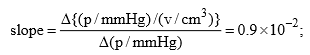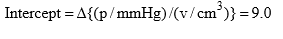Form the Langmuir equation, we will have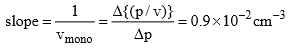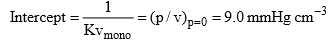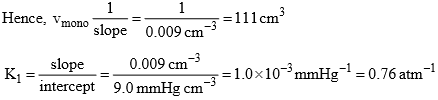(i) The fraction of surface covered at the given pressures are as follows:

 p/mmHg 100 200 300 400 500 600 700 v/cm3 10.2 18.6 25.5 31.4 36.4 41.6 46.1 θ = v/vmono 0.092 0.168 0.23 0.283 0.332 0.375 0.415

(iii) The change of standard free energy on adsorption at 273 K will be given by ΔadsG° = –RT ln (K1/atm–1) = –8.314 JK–1 mol–1) (273 K) (2.303) (log 0.76)

10. Types of Adsorption Isotherms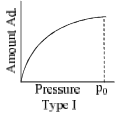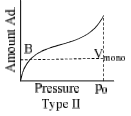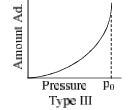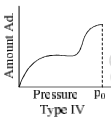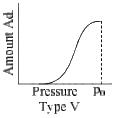The relationship at a given temperature between the amount of the gas adsorbed equilibrium pressure is known as an adsorption isotherm.

• Type I isotherm show a fairly rapid rise in the amount of adsorption with increasing pressure upto a limiting value (Langmuir-type isotherm). Example adsorption of H2 and N2 on charcoal at –180°C.
• Type II isotherms represent multilayer physical adsorption on non-porous solids. Point B represents the formation of an adsorbed monolayer. Example adsorption of N2 on silica gel.
• Type III isotherms show amount of adsorption keeps on rising in each case with increase in pressure. Example adsorption of Br2 on silica or alumina get at 80°C.
• Type IV and type V are observed in those cases where there is capillary condensation in porous solids. Example of type IV: adsorption of benzene on silica gel at 50°C and example of type V: adsorption of water vapour on activated carbon at 100°C.

Relation between adsorption and energy of liquefaction of the gaseous adsorbate more readily soluble and easily liquefiable gases such as NH3, HCl, Cl2 and SO2 are adsorbed more than so called permanent gases like H2, N2 and O2.

Offer running on EduRev: Apply code STAYHOME200 to get INR 200 off on our premium plan EduRev Infinity!

## Physical Chemistry

64 videos|92 docs|31 tests

,

,

,

,

,

,

,

,

,

,

,

,

,

,

,

,

,

,

,

,

,

;